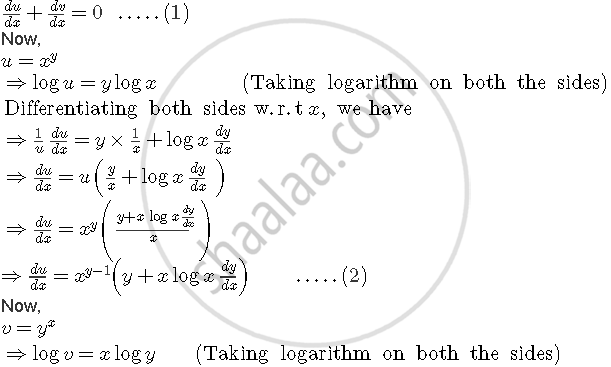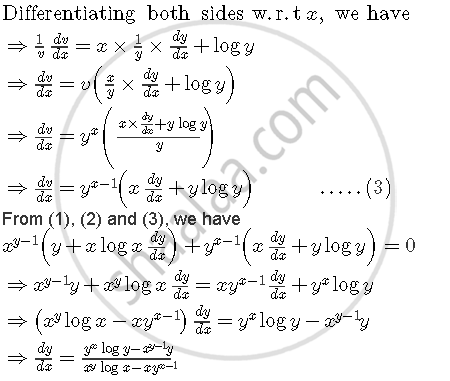Share

# if xx+xy+yx=ab, then find dy/dx. - CBSE (Science) Class 12 - Mathematics

#### Question

if xx+xy+yx=ab, then find dy/dx.

#### Solution 1

xx+xy+yx=ab........(i)

Let u=x^x

log u=xlogx

1/u*(du)/dx=x * 1/x+logx

therefore (du)/dx=x^x(1+logx)

Let v=x^y

logv =ylogx

1/v (dv)/dx=(y/x+logx dy/dx)

therefore (dv)/dx=x^y(y/x+logx dy/dx)

Let w=y^x

logw=x log y

1/w.(dw)/dx=(x/y*dy/dx+logy)

therefore (dw)/dx=y^x(logy+x/y*dy/dx)

(i) can be written as

u + v + w = ab

du/dx+dv/dx+dw/dx=0

=>x^x+x^xlogx+x^yy/x+x^y logx dy/dx+y^xlogy+y^x x/y dy/dx=0

=>dy/dx(x^ylogx+y^x x/y)=x^x+x^xlogx+x^y y/x+ y^x logy

=> dy/dx (x^y*logx+xy^(x-1))=(x^x+x^xlogx+yx^(y-1)+y^x*logy)

therefore dy/dx=(x^x+x^xlogx+yx^(y-1)+y^x*logy)/(x^y*logx+xy^(x-1))

#### Solution 2

Let u = xy and v = yx

Then, u + v = ab

Differentiating both sides w.r.t x, we getIs there an error in this question or solution?

#### Video TutorialsVIEW ALL 

Solution if xx+xy+yx=ab, then find dy/dx. Concept: Logarithmic Differentiation.
S